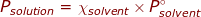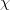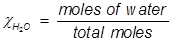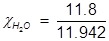# Vapor Pressure Formula

Vapor Pressure Formula

When a liquid evaporates, the gaseous molecules created escape into the air. If the liquid is in a closed container, the gaseous molecules created will not escape but remain above the liquid. These evaporated particles created a pressure above the liquid, this is known as the vapor pressure.

If a solid is dissolved into the liquid, a solution is created. The vapor pressure of the solution is lowered by the addition of the solute. Raoult’s law explains how the vapor pressure of a liquid is altered by the addition of a solute.Psolution = the vapor pressure of the solutionsolvent = the mole fraction of the solvent in the solution

P°solvent = the vapor pressure of the pure solvent at standard conditions

Vapor Pressure Formula Questions:

1. What is the vapor pressure of a solution at 25°C containing 3.5 moles of glucose in 10.0 moles of water? (the vapor pressure of pure water at 25°C is 23.8 torr)

In order to solve for Raoult’s law, the mole fraction of water must be obtained.H2O=0.741;

Using the mole fraction of water, solve for Raoult’s law.Psolution=0.741 x 23.8 torr

Psolution=17.6 torr

2. What is the vapor pressure of a solution at 25°C when 25.5 grams of glucose (C6H12O6) is dissolved in 212 grams of water? (the vapor pressure of pure water at 25°C is 23.8 torr)

In order to solve for the mole fraction of water, both the grams of glucose and grams of water must be converted to moles.Now solve for the mole fraction of water.H2O=0.988;

Finally, solve for the partial pressure of the solution.Psolution=0.988 x 23.8 torr

Psolution=23.5 torr

 Related Links: Gases: Pressure Quiz Pneumatics Examples Pressure Formula Gay-Lussac's Law Formula Boyle's Law Formula Osmotic Pressure Formula Ideal Gas Law Formula